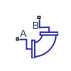# Elbow (IL)

Pipe turn in an isothermal liquid network

Since R2020a

•Libraries:
Simscape / Fluids / Isothermal Liquid / Pipes & Fittings

## Description

The Elbow (IL) block models flow in a pipe turn in an isothermal liquid network. Pressure losses due to pipe turns are calculated, but the effect of viscous friction is omitted.

Two Elbow type settings are available: `Smoothly-curved` and ```Sharp-edged (Miter)```. For a smooth pipe with a 90o bend and modeled losses due to friction, you can also use the Pipe Bend (IL) block.

### Loss Coefficients

When the parameter is ```Smoothly curved```, the block calculates the loss coefficient as:

`$K=30{f}_{T}{C}_{angle}.$`

The block calculates Cangle, the angle correction factor, from Keller  as

`${C}_{angle}=0.0148\theta -3.9716\cdot {10}^{-5}{\theta }^{2},$`

where θ is the value of the Bend angle parameter in degrees. The block defines the friction factor, fT, as the value for clean commercial steel. The block then interpolates the values from tabular data based on the internal elbow diameter for fT provided by Crane . This table contains the pipe friction data for clean commercial steel pipe with flow in the zone of complete turbulence.

Nominal size½"¾"1"1 ¼"1 ½"2"2 ½, 3"4"5"6"8-10"12-16"18-24"
Friction factor, fT.027.025.023.022.021.019.018.017.016.015.014.013.012

The values provided by Crane are valid for diameters up to 600 millimeters. The friction factor for larger diameters or for wall roughness beyond this range is calculated by nearest-neighbor extrapolation.

When the parameter is ```Sharp-edged (Miter)```, the block calculates the loss coefficient K for the bend angle, α, according to Crane :### Mass Flow Rate

Mass is conserved through the pipe segment:

`${\stackrel{˙}{m}}_{A}+{\stackrel{˙}{m}}_{B}=0.$`

The mass flow rate through the elbow is calculated as:

`$\stackrel{˙}{m}=A\sqrt{\frac{2\overline{\rho }}{K}}\frac{\Delta p}{{\left[\Delta {p}^{2}+\Delta {p}_{crit}^{2}\right]}^{1/4}},$`

where:

• A is the flow area.

• $\overline{\rho }$ is the average fluid density.

• Δp is the pipe segment pressure difference, pApB.

The critical pressure difference, Δpcrit, is the pressure differential associated with the Critical Reynolds number, Recrit, the flow regime transition point between laminar and turbulent flow:

`$\Delta {p}_{crit}=\frac{\overline{\rho }}{2}K{\left(\frac{\nu {\mathrm{Re}}_{crit}}{D}\right)}^{2},$`

where

• ν is the fluid kinematic viscosity.

• D is the elbow internal diameter.

### Energy Balance

The block balances energy such that

`${\Phi }_{A}+{\Phi }_{B}=0,$`

where:

• ϕA is the energy flow rate at port A.

• ϕB is the energy flow rate at port B.

## Ports

### Conserving

expand all

Liquid entry or exit port to the pipe segment.

Liquid entry or exit port to the pipe segment.

## Parameters

expand all

Bend specification of the pipe segment. A sharp-edged, or mitre, bend introduces a sharp change in flow direction, such as at a pipe joint, and the flow losses are modeled by a separate set of empirical data from gradually-turning pipe segments.

Internal diameter of the pipe elbow segment.

Angle of the swept pipe curve.

Upper Reynolds number limit for laminar flow through the pipe segment.

 Crane Co. Flow of Fluids Through Valves, Fittings, and Pipe TP-410. Crane Co., 1981.

 Keller, G. R. Hydraulic System Analysis. Penton, 1985.